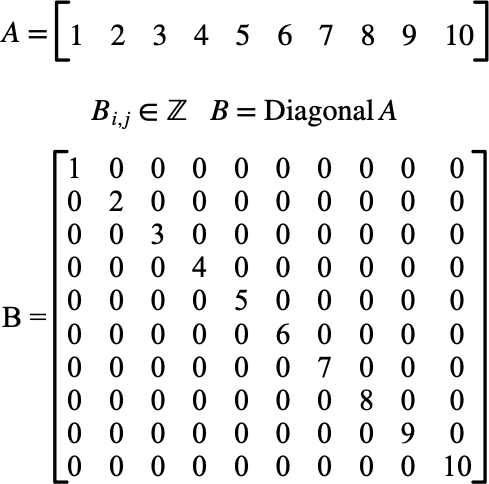# $$\text{Diagonal}$$¶

You can use the $$\text{Diagonal}$$ function to return a diagonal matrix from supplied values in another matrix.

You can use the \diagonal backslash command to insert this function.

The following variants of this function are available:

• $$\text{matrix } \text{Diagonal} \left ( \text{<matrix>} \right )$$

The returned matrix will be the same type as $$\text{<matrix>}$$. The returned value will be a column matrix with one entry per diagnonal entry in the the matrix.

Figure 123 shows the basic use of the $$\text{DiagonalEntries}$$ function.Figure 123 Example Use of The Diagonal Function